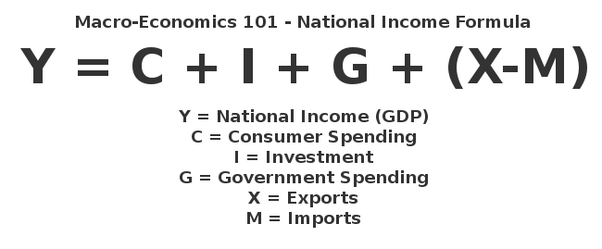# Variables, values, inputs, outputs¶

## Use variables to store values¶

• Variables are names for values.

• In Python the `=` symbol assigns the value on the right to the name on the left.

• The variable is created when a value is assigned to it.

• Here, Python assigns an age to a variable `age` and a name in quotes to a variable `first_name`.

```age = 42
first_name = 'Ahmed'
```
• Variable names

• can only contain letters, digits, and underscore `_` (typically used to separate words in long variable names)

• are case sensitive (age, Age and AGE are three different variables)

• Variable names that start with underscores like `__alistairs_real_age` have a special meaning so we won’t do that until we understand the convention.

## Use `print` to display values.¶

• Python has a built-in function called `print` that prints things as text.

• To add a string to the printout, wrap the string in single or double quotes.

Tip

The values passed to the function are called arguments

```print(first_name, 'is', age, 'years old')
```
• `print` automatically puts a single space between items to separate them.

• And wraps around to a new line at the end.

## Variables must be created before they are used.¶

• If a variable doesn’t exist yet, or if the name has been mis-spelled, Python reports an error. (Unlike some languages, which “guess” a default value.)

```print(last_name)
```
• The last line of an error message is usually the most informative.

• We will look at error messages in detail later.

## Variables Persist Between Cells¶

Be aware that it is the order of execution of cells that is important in a Jupyter notebook, not the order in which they appear. Python will remember all the code that was run previously, including any variables you have defined, irrespective of the order in the notebook. Therefore if you define variables lower down the notebook and then (re)run cells further up, those defined further down will still be present. As an example, create two cells with the following content, in this order:

```print(myval)
```
```myval = 1
```

Note

If you execute this in order, the first cell will give an error. However, if you run the first cell after the second cell it will print out `1`. To prevent confusion, it can be helpful to use the `Kernel` -> `Restart & Run All` option which clears the interpreter and runs everything from a clean slate going top to bottom.

## Use variables in calculations¶

```age = age + 3
print('Age in three years:', age)
```

## Basic GDP calculation¶

The following code implemens a simple macroeconomics equation. Run it on your python environment.

```C = 1000000
I = 500000
G = 800000
X = 100000
M = 2000000
Y = C + I + G + (X - M)
print(Y)
```Exercise: calculate yearly GDP of a country given certain facts

Copy and paste the code bellow in your jupyter notebook. Complete the empty spaces in the cell bellow to calculate the gdp considering the given GDP formula above.

Assume that Consumer spending is 1 million per year, Investment is 500 K, Government spending is 800 K, Esports is 100 K and imports 2 million.

```def get_gdp(_,_,_,_):
return  _ + _ + _ + (_ - _)

island_gdp = get_gdp(1000000,500000,800000,100000,2000000)
print(island_gdp)
```
```# Run this code in your computer
import this
```

Exercise: Do a little code review

After reading The Zen of Python, write in the comments why this code:

```def get_gdp(_,_,_,_):
return  _ + _ + _ + (_ - _)
```

might be better than this:

```C = 1000000
I = 500000
G = 800000
X = 100000
M = 2000000
Y = C + I + G + (X - M)
```